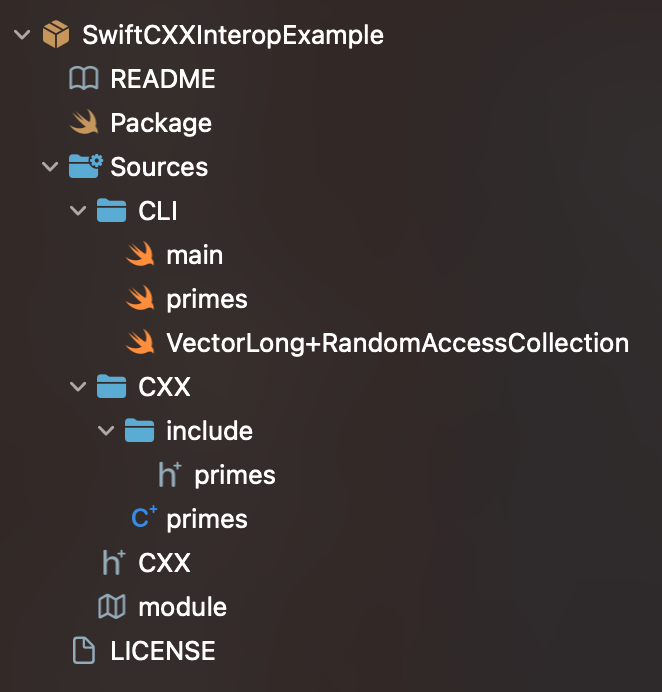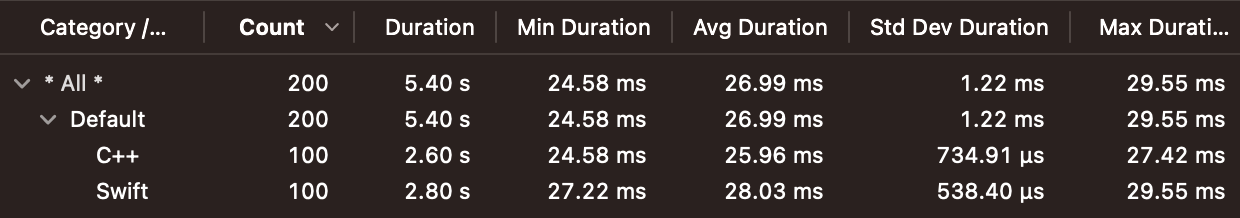Articles about Swift development, by Konstantin Semianov

# Getting started with Swift-C++ interop

Playing around with Swift toolchain experimental features.

## Intro

Swift is a very comfortable language. It has some quirks and a learning curve, but ultimately you can ship production-ready code with it pretty fast. However, sometimes you have performance-critical sections and Swift just doesn't cut it. In such cases, a popular choice is using C++. And the question arises "how do I call this C++ func from Swift"? Usually, you have to write an Objective-C wrapper that will act as a public interface for your C++ code. And Swift toolchain can import Objective-C declarations to Swift. The main limitation is that you cannot use C++ classes in Objective-C, only simple POD structs.

We'll write a Sieve of Eratosthenes algorithm with both C++ and Swift. Then learn how to enable C++ interop, call C++ code from Swift, and compare implementation performance. Keep in mind that the feature is experimental and subject to changes. This publication compiles on Xcode Version 14.2

## Algorithm

The Sieve of Eratosthenes finds all prime numbers less than or equal N. Prime number is an integer that's divisible only by itself and 1. The algorithm creates a boolean array to indicate if each number is prime. And progressively iterates over them, marking all multiples as not prime.

Here is the Swift implementation.

``````// primes.swift

func primes(n: Int) -> [Int] {
var isPrime = [Bool](repeating: true, count: n + 1)

for value in stride(from: 2, to: n + 1, by: 1) where isPrime[value] {
if value * value > n { break }

for multiple in stride(from: value * 2, to: n + 1, by: value) {
isPrime[multiple] = false
}
}

var result = [Int]()

for value in stride(from: 2, to: n + 1, by: 1) where isPrime[value] {
result.append(value)
}

return result
}
``````

For C++ we need a header and a source file. Note, that we `typedef` to have a cleaner name for referring to `std::vector<long>`.

``````// primes.hpp

#include <vector>

typedef std::vector<long> VectorLong;

VectorLong primes(const long &n);
``````
``````// primes.cpp
#include <algorithm>

#include "primes.hpp"

VectorLong primes(const long &n) {
std::vector<char> isPrime(n + 1); // faster than std::vector<bool>
std::fill(isPrime.begin(), isPrime.end(), true);

for (long value = 2; value * value <= n; ++value) {
if (!isPrime[value]) { continue; }

for (long multiple = value * 2; multiple <= n; multiple += value) {
isPrime[multiple] = false;
}
}

VectorLong result;

for (long value = 2; value <= n; ++value) {
if (!isPrime[value]) { continue; }

result.push_back(value);
}

return result;
}
``````

## Project structureWe'll do a Swift Package with two separate targets to hold our Swift and C++ code. To import C++ code from Swift, we need a modulemap.

``````// module.modulemap

module CXX {
requires cplusplus
}

// CXX.hpp

#include "primes.hpp"
``````

And do not forget to pass `-enable-experimental-cxx-interop` to the Swift target in `Package.swift`.

``````// swift-tools-version: 5.7

import PackageDescription

let package = Package(
name: "SwiftCXXInteropExample",
platforms: [
.macOS(.v12),
],
products: [
.library(name: "CXX", targets: ["CXX"]),
.executable(name: "CLI", targets: ["CLI"])
],
dependencies: [],
targets: [
.target(name: "CXX"),
.executableTarget(
name: "CLI",
dependencies: ["CXX"],
swiftSettings: [.unsafeFlags(["-enable-experimental-cxx-interop"])]
)
]
)
``````

See the doc from Apple for more info on how to enable C++ interop.

## Trying out

It's much easier to use our `VectorLong` from Swift with conformance to `RandomAccessCollection` and, happily, it's really easy to do.

``````import CXX

extension VectorLong: RandomAccessCollection {
public var startIndex: Int { 0 }
public var endIndex: Int { size() }
}
``````

Now we can call our C++ function from Swift and print the results to the console.

``````let cxxVector = primes(100)
let swiftArray = [Int](cxxVector)
print(swiftArray)
``````

Let's see if our C++ implementation actually performs faster.

``````let signposter = OSSignposter()

let count = 100
let n = 10_000_000

for _ in 0..<count {
let state = signposter.beginInterval("C++")
let _ = primes(n)
signposter.endInterval("C++", state)
}

for _ in 0..<count {
let state = signposter.beginInterval("Swift")
let _ = primes(n: n)
signposter.endInterval("Swift", state)
}
``````Slightly faster with an average duration of 26 ms vs. 28 ms for Swift.

## Final thoughts

We were able to directly use `std::vector` in Swift. I personally haven't found a convenient way to round-trip between Swift `Map` and `std::map`, `Set` and `std::set`. Nevertheless, C++ interop is rapidly developing and the future seems bright.

CppInteroperability folder in the Swift repo contains more information on interop features, limitations and plans.

See the full code at https://github.com/ksemianov/SwiftCXXInteropExample